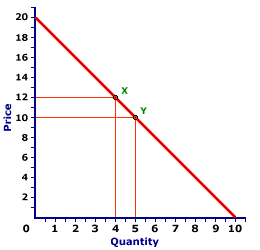Friday  October 22, 2021
 AmosWEB means Economics with a Touch of Whimsy!ABSOLUTE POVERTY LEVEL: The amount of income a person or family needs to purchase an absolute amount of the basic necessities of life. These basic necessities are identified in terms of calories of food, BTUs of energy, square feet of living space, etc. The problem with the absolute poverty level is that there really are no absolutes when in comes to consuming goods. You can consume a given poverty level of calories eating relatively expensive steak, relatively inexpensive pasta, or garbage from a restaurant dumpster. The income needed to acquire each of these calorie "minimums" vary greatly. That's why some prefer a relative poverty level.ENDPOINT ELASTICITY FORMULA:

A simple technique for calculating the coefficient of elasticity by estimating the elasticity for discrete changes in two variables using the initial values of each. The distinguishing characteristic of this formula is that percentage changes are calculated based on the initial values of each variable. An alternative technique is the midpoint elasticity formula.
The endpoint elasticity formula is a simple, first-approximation, method of calculating elasticity, especially the price elasticity of demand, price elasticity of supply, income elasticity of demand, and cross elasticity and demand. This formula is most often used at the introductory level of economic instruction.

### The Formula

The endpoint elasticity formula for calculating the response of changes in B to changes in A is given as:

 endpointelasticity = (B2 - B1)B1 ÷ (A2 - A1)A1
The first term on the right-hand side of the equation is the percentage change in variable B. The second term is the percentage change in variable A. The individual items are interpreted as this: A1 is the initial value of A before any changes, A2 is the ending value after A changes, B1 is the initial value of B before any changes, and B2 is the ending value after B changes.

The numerator of each term on the right-hand side of the equation [(A2 - A1) and (B2 - B1)] is the discrete change in A and B, respectively. The denominator of each term, A1 and B1, is the base value from which the percentage change is calculated.

### An Simple Example

A Standard Demand CurveThe time has come for a numerical example to illustrate. The demand curve to the right should help. Suppose the demand price of Wacky Willy Stuffed Amigos (those cute and cuddly armadillos and tarantulas) INCREASES from \$10 to \$12. This price increase causes the quantity demanded to decrease from 5 to 4 Stuffed Amigos. Using the endpoint elasticity formula, the price increases by 20 percent, a \$2 increase from a \$10 initial price. This method also results in a 20 percent decrease in the quantity demanded, a decline of 1 Stuffed Amigo from an initial value of 5. This seems relatively simple and straightforward. The price elasticity of demand using the endpoints elasticity formula is thus equal to 1.

Or is it?

### An Endpoint Problem

The problem with the endpoints elasticity formula for demand elasticity is that the coefficient of elasticity depends on whether price increases or decreases. This is demonstrated by asking the question: What is the coefficient of elasticity for this same range of the demand curve, if the price DECREASES from \$12 to \$10 causing the quantity demanded to increase from 4 to 5? In this case, the change in price is 16.7 percent, a \$2 decrease from a \$12 initial price, and the change in quantity is 25, an increase of 1 Stuffed Amigo from an initial value of 4. This results in a coefficient of elasticity of 1.5.

Over the same segment of the demand curve, a price INCREASE indicates a different coefficient of elasticity than does a price DECREASE. This can become confusing.

This problem is prevented by using an alternative method of calculating the coefficient of elasticity, the midpoint elasticity formula. This alternative uses the average of the beginning and ending values of each variable (that is, the midpoint) as the base value for calculating percentage changes. If the discrete changes are relatively small, then the two methods are approximately the same.

 <= EMPLOYMENT RATE ENERGY PRICES, AGGREGATE SUPPLY DETERMINANT =>Recommended Citation:

ENDPOINT ELASTICITY FORMULA, AmosWEB Encyclonomic WEB*pedia, http://www.AmosWEB.com, AmosWEB LLC, 2000-2021. [Accessed: October 22, 2021].

Check Out These Related Terms...

Or For A Little Background...

And For Further Study...
Search Again?GRAY SKITTERY[What's This?] Today, you are likely to spend a great deal of time browsing about a thrift store seeking to buy either a coffee cup commemorating the first day of spring or a printer that works with your stockpile of ink cartridges. Be on the lookout for mail order catalogs with hidden messages.Your Complete ScopeAl Capone's business card said he was a used furniture dealer."A stumble may prevent a fall. "-- Margaret Thatcher, British prime ministerACRSAccelerated Cost Recovery SystemA PEDestrian's Guide Xtra CreditTell us what you think about AmosWEB. Like what you see? Have suggestions for improvements? Let us know. Click the User Feedback link.| | | | | | | | | | |
| | | |

Thanks for visiting AmosWEB